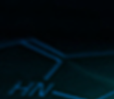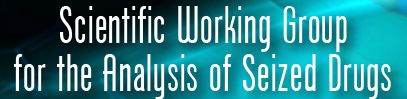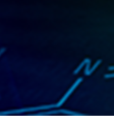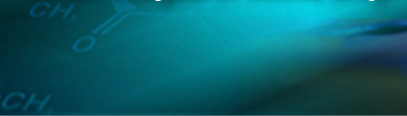IV. ANALYTICAL TECHNIQUES

4. Gas Chromatography (GC) including Gas Chromatography/Mass Spectrometry (GC/MS)

1. Draw a schematic diagram of a gas chromatograph. Briefly explain the function of each of the main components.
2. Briefly describe in non-technical language the theory and operation of a gas chromatograph.
3. How does an FID detector work?
4. Describe the physical and chemical characteristics of a capillary column.
5. What is a van Deemter plot? What valuable property does a van Deemter plot illustrate?
6. Compare the advantages and disadvantages for the use of nitrogen, helium and hydrogen as a carrier gas.
7. Why is helium or hydrogen the main carrier gas used for capillary columns?
8. Define the terms “adsorption”, “absorption” and “partition”. Explain the differences.
9. Define the terms “effective number of theoretical plates” and “height equivalent to a theoretical plate. Which parameter is a better measure of the performance of a given column and why?
10. What are the differences between an isothermal and a programmed temperature gas chromatography (PTGC) method? When might a PTGC method be used?
11. What are the advantages and disadvantages to a programmed temperature method?
12. What is the difference between a split and a split-less injection?
13. When is one injection technique employed over the other?
14. Which is the most common detector employed with gas chromatography in forensic laboratories? Why?
15. What symptoms would indicate time for a septum change on a gas chromatograph?
16. Why might a substance produce an asymmetric peak in a gas chromatogram?
17. Offer reasons for a tailing peak versus a fronting peak.
18. How might the peak shape be improved?
19. What are some of the possible causes of a GC that does not show any peaks in the chromatogram after sample injection?
20. Methane is injected on a HP-1 column with the dimensions of 15 m length x 0.32 mm internal diameter x 0.25 um film thickness operating at an oven temperature of 110 C using helium as the carrier gas. If methane elutes from the column at a retention time of 0.70 minutes, what are the linear velocity (µ) and the flow rate (F) of the carrier gas?
21. What steps does your laboratory take to ensure that the GC-FID instruments are operating correctly? Do these steps meet or exceed LOM requirements?
22. Why is it important to base extract a TFAP – Methamphetamine derivatization reaction prior to injecting the solution on the gas chromatograph?
23. Draw the chemical structure of the following polymeric stationary phases: 100% dimethylpolysiloxane (HP-1, DB-1. etc.) and (5%-phenyl)-methylpolysiloxane (HP-5, DB-5, etc.). Note the relative polarity of the phase and the temperature limit of a typical column coated with the phase.
24. You are looking at two different chromatograms of the same sample. You notice that the area of one is 5000 and the other is 8000. If using the split injection technique, which of the following parameters was most likely different? Sample injection amount, Column flow, Split ratio, Both A and C.
25. True or False? Molecules with a great affinity for the stationary phase spend less time in that phase and consequently take less time to reach the detector.
26. True or False? Split-less injection is normally used when there is an abundance of sample.
27. True or False? The FID responds proportionately to the number of --CH2-- groups introduced into the flame.
28. True or False? The major advantage of the internal standard technique is that the exact size of injection does not need to be known.
29. True or False? One problem with temperature programming is that the back-pressure increases with temperature and will reduce carrier flow if a flow controller is not used.
30. What elements should be addressed when preparing a sample for GC analysis?
31. What problem may arise if GC samples are not routinely filtered?
32. What is meant by split injection?
33. What is the effect of a column’s liquid phase as it gets thinner/thicker?
34. What is the role of the septum?
35. What is meant by un-retained peak? Discuss in context of liquid and gas phases.
36. What are advantages of isothermal and temperature programs?
37. If the flow is increased, retention time ________.
38. Does head pressure affect retention time?
39. Define retention time.
40. Describe a quality tool that reduces carry over.
41. A blank has cocaine at the same retention time as a standard. Troubleshoot.
43. What is the role of the filament? Why is the voltage set at 70 eV?
44. You do not have emission current. What are the primary causes of this, and what could be done to verify this?
45. Define base peak and molecular ion.
46. An amine standard is injected but no molecular ion is detected. Explain.
47. Describe how GC works in theory. How would you describe this to a lay jury? (Assume no more than a high-school level of chemistry.)
48. List three types of GC detectors. What sorts of analytes are they designed for?
49. What is derivatization?
50. Why is this sometimes necessary in drug analysis?
51. What are the drawbacks to derivatization?
52. Describe how GC can be used as a qualitative technique
53. Describe how GC and be used as a quantitative technique.
54. List the most common preventative maintenance issues associated with GCMS.
55. List some issues that can cause retention time to shift.
56. Define the following terms: EI, CI, base peak, molecular ion, m/z, amu.
57. What is the purpose of a mass analyzer?
58. What is a magnetic sector mass spectrometer?
59. What is a time of flight mass spectrometer?
60. What is a quadrupole mass spectrometer?
61. What is an ion trap mass spectrometer?
63. What is fragmentation?
64. What are the four basic inlet systems used in mass spectrometers?
65. What are the common fragmentation ions of a primary, secondary or tertiary amine in the amphetamine family?
66. When would a derivatization be necessary? What should be considered in the derivative selection?
67. What is the most common instrumental mass spectral technique for the determination of molecular weight?
68. Besides EI and CI, name two other useful ionization techniques.
69. What are the calibration and maintenance procedures for the GC-MS used in your laboratory?
70. Name the calibration compound used to “tune” the EI mass spectrometer. Identify the three major fragment ions used in the calibration tune.
71. Explain the function of each of the following parts of the EI mass spectrometer: ion source, repeller, lens stack (draw out lens, ion focus, entrance lens), quadrupole (aka mass filter), electron multiplier.
72. What are the drawbacks to setting the threshold on the mass spectrometer too low?
73. What are the four classes of mass filters used to select and filter ions?
74. What three problems can be attributed to leaks in the GC-MS system?
75. If the GC-MS presents an error message stating that there is no emission current, what might this indicate?
76. What type of ionization do the mass spectrometers in your laboratory employ?
77. What could happen at a voltage of less than 70eV?
78. How often should the tune be performed?
79. How often should the injection port liner be changed?
80. How would you know if there was air or water in the system?
81. What are the base peaks and molecular ions are for each of the following: cocaine, heroin, phencyclidine, LSD, methamphetamine?
82. Tien-Lai Li et al have shown that when underivatized methamphetamine HCl in methanol (or ethanol) is analyzed by GC-MS with old inlet liners at temperatures above 200C, appreciable amounts of N,N-Dimethylamphetamine (DMA) and amphetamine are produced. What steps do they recommend to avoid this?
83. List some different types of MS detectors, and briefly describe the positives and drawbacks of each.
84. List some different types of ionization techniques used in MS. What is the primary one used in drug analysis?
85. Describe how you would explain MS theory to a lay jury.
86. Explain why MS is considered a category A technique by SWGDRUG.
87. List some practices that minimize contamination during GC-MS analysis.
88. Why is this of critical importance for drug analysis?
89. You have a mixture of two analytes that co-elute on your standard GCMS program. Without relying on data analysis/software techniques, list some things that you could do to improve their baseline separation.
90. Describe how MS can be used quantitatively. List some reasons why this would be superior to other quantitative techniques, and some drawbacks to its use.
91. List some resources that could be used in the identification of an unknown mass spectrum.
92. What structural information can you get from MS analysis?
93. What types of analytes would not lend themselves to analysis via MS?
94. Will GC-MS distinguish dextropropoxyphene from dl- or l- propoxyphene? Explain.
95. Will GC-MS distinguish d-pseudoephedrine from dl- or l-pseudoephedrine? Explain.
96. As a minimum, the following terms should be understood: chromatography, stationary phase, mobile phase, peak, baseline, peak-widths, peak base, peak area, peak maximum, peak height, tailing, fronting, column volume, extra-column volume (sometimes called dead-volume), hold-up (the retention time of an unretained peak), total retention time, adjusted retention time, retention factor (sometimes called capacity factor), relative retention, separation factor, detector.
97. List the quality control measures that you are responsible for when operating the GCMS.
98. Here is a list of some common problems encountered when operating the GC/MS. Give a short description of the possible causes of the problem and the measures you would undertake to resolve the problem: peaks in solvent blank, unlabeled peaks in a standard mix, blank and standard responses too low, no emission current, low or no ions in the tune, normal standard response but with very high background, 28 and 32 ions in standard tune/tune report, Electron multiplier volts very high in standard tune/tune report, poor heroin response in a standard mix, poor morphine response in a standard mix.
99. What are some of the problems of analyzing carbamates?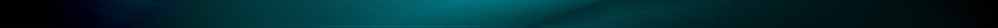Last Update June 2022